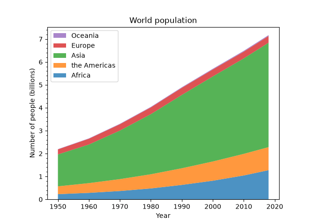# matplotlib.axes.Axes.stackplot¶

Axes.stackplot(axes, x, *args, labels=(), colors=None, baseline='zero', data=None, **kwargs)

Draw a stacked area plot.

Parameters: x(N,) array-like y(M, N) array-likeThe data is assumed to be unstacked. Each of the following calls is legal: stackplot(x, y) # where y has shape (M, N) stackplot(x, y1, y2, y3) # where y1, y2, y3, y4 have length N  baseline{'zero', 'sym', 'wiggle', 'weighted_wiggle'}Method used to calculate the baseline: 'zero': Constant zero baseline, i.e. a simple stacked plot. 'sym': Symmetric around zero and is sometimes called 'ThemeRiver'. 'wiggle': Minimizes the sum of the squared slopes. 'weighted_wiggle': Does the same but weights to account for size of each layer. It is also called 'Streamgraph'-layout. More details can be found at http://leebyron.com/streamgraph/. labelslist of str, optionalA sequence of labels to assign to each data series. If unspecified, then no labels will be applied to artists. colorslist of color, optionalA sequence of colors to be cycled through and used to color the stacked areas. The sequence need not be exactly the same length as the number of provided y, in which case the colors will repeat from the beginning. If not specified, the colors from the Axes property cycle will be used. **kwargsAll other keyword arguments are passed to Axes.fill_between. list of PolyCollectionA list of PolyCollection instances, one for each element in the stacked area plot.

Notes

Note

In addition to the above described arguments, this function can take a data keyword argument. If such a data argument is given, every other argument can also be string s, which is interpreted as data[s] (unless this raises an exception).

Objects passed as data must support item access (data[s]) and membership test (s in data).

## Examples using matplotlib.axes.Axes.stackplot¶